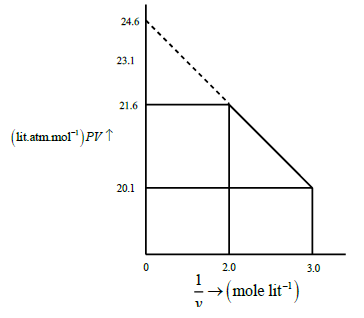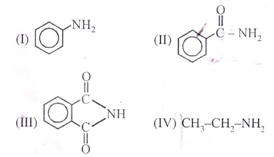# Chemistry Online Test for JEE, NEET and EAMCET Exams

1. Phenol can be distinguished from ethanol by the following reagents except

2. The rate of a first order reaction is 1.5 ×10-2
mol L-1 min-1 at 0.5 M concentration of the reactant. The
half life of the reaction is

3. Which of the following is used as “morning after pill”?

4. For the given complex ,[CoCl2(en)(NH3)]+ the number of geometrical isomer’s, the number of optical isomers and the total number of isomers of all type
possible respectively are

5. The correct order of EM+2/M values with negative sign for the four successive elements Cr,Mn,Fe and Co is

6. 51. Cyclohexene on ozonolysis followed by reaction with Zn dust and water gives compound ‘E’. Compound ‘E’ on further treatment with aqu.KOH yields compound ‘F’. Compound ‘F’ is

7. What would be the product formed when 1-bromo-3 chloro cyclo butane reacts with two equivalents of metallic sodium in ether

8. In  Na2O having antifluorite structure

9. A solution ‘X’ contains 30 mole percent of A and is in equilibrium with its vapour that contains 40 mole percent of ‘B’. The ratio of vapor pressure of pure A and B will be:

10. The standard reduction potential for  Cu+2/ Cu  is +0.34V.Calculate the reduction potential at pH=14
for the above couple.   Ksp of Cu(OH)2= 1.0×10-19

12. When the value of azimuthal quantum number is 3, the maximum and the minimum values of the spin multiplicities are

13. The weight of H2O2present in 80 ml of 10V H2O2 solution is

14. If mercury is used as cathode in the electrolysis of aqueous NaCl solution, the ions discharged at cathode are

15. For one mole of a vanderwaal’s gaswhen b=0 and T=300K, the PV vs1/V plot is shown below. The value of vander waal’s constant ‘a’ in atm.lit2 mole-2 is (graph not to scale)16. The equivalent weight of HCl in the given reaction is
KCr2O7 +14 HCl -->2KCl + 2CrCl3 +3Cl2 +H2O

17. At what temperature will average speed of the molecules of the second member of the series  CnH2n be the same of Cl2 at 6270C?

18. When two moles of an ideal gas (Cpm=(5/2)R) heated from 300K to 600K at constant pressure. The change in entropy of gas (∆s ) is

19. I2(aq) + I- (aq) --> I-3(aq)+  We started with 1 mole of I2 and 0.5 mole of I- in one litre flask. After equilibrium is reached excess of AgNO3 gave 0.25 mole of yellow precipitate. Then
Equilibrium constant is

20. The values of electro negativity of atoms A and B are 1.20 and 4.0 respectively. The percentage ionic character of A-B bond is

21. The plots of 1/ XA Vs 1/YA ( where X and YA are the mole fraction of liquid A in liquid and vapour phase respectively) is linear with slope and intercepts respectively

22. An oxide of Nitrogen which form reddish brown fumes in air is

23. Iron sulphide is heated in air to form ‘A’ . ‘A’ is dissolved in water to give ‘Y’. The basicity of ‘Y’ is

24. Which one of the following oxyacids of chlorine is the least
oxidising in nature

25. Which of the following organ metallic compound is σ and π
bonded?

27. An acid-base indicator has a In Kln of 3 ×10-5. The acid form of indicator is red and the basic form is blue.
Then

28. Which of the following vitamin contains ionone ring and
hydrocarbon chain.

29. Which of the substances added to soap make it antiseptic?

Incorrect statements about X and Y are

32. The correct order of increasing acidic strength of the compounds
A) CH3CO2H
B) MeOCH2CO2H
C) CF3CO2H

The ratio of number of moles of Hydrogen atoms required to get 1 mole of azobenzene and 1 mole of hydrazobenzene is

34. A possitive carbylamine test is given by

35. Which of the following amines form N-nitroso derivative when treated with NaNO2 and HCl

36. Which of the following compound react faster through SN2mechanism

37. The most volatile alkane is

38. The hybridisation of Beryllium in Beryllate ion formed when Beryllium hydroxide dissolves in excess of alkali?

39. The correct statement among the following is:
i) NaBH4 produce BH6 on reaction with I2

ii) Reaction between B2H6 and NMe3 is an acid base reaction

iii) BH6 is produced on industrial scale by reaction with BF3, NaH

iv) Boranes on hydrolysis produce
B2H6 and H2 gas

40. Incorrect statement among the following is

41. Which of the following will have highest negative enthalpy of formation?

42. Most basic and acidic oxides are formed by the following 3rd period elements.

43. The pair of molecules having same geometry and same bond angles are

44. Kjeldahl’s method cannot be used for estimation of nitrogen in
I) C6H5CO NH2  II) Pyridine
III)  C6H5-N=N-C6H5

45. Benzene reacts with cholorine in the presence of U.V. light and gives

46. If lone pair of electron occupy axial position then, incorrect about is :

47. Which of the following is incorrect for the indicated property

48. Select species which is planar at nitrogen

49. Total number of coordination isomers shown by complex  [Co(NH3)6] [Cr(Cl)6] is:

50. Xe has max. EN in:-

51. The correct statement/s about H2 S2 O7 is/are

52. Find correct statement

53. The first ionization potential of Na is 5.1eV The value of electron gain enthalpy of Na+ will be

54. Which one of the following is a mineral of iron?

55. In which of the following pairs, both the species are not isostructural?

56. Which property is common in diamond and graphite

57. When H2S is passed through acidified KMnO4  we get

58. The shortest wavelength of He+ ion in Balmer series is x, then longest wavelength in the paschen series of Li2+is

59. The molecule which is having maximum number of lone pair

60. What will be the equivalent weight of H3PO3 in the following reaction. 4H3PO3 → 3H3PO4 +PH3

61. 20g of naphthoic acid (C11H8O2) is dissolved in 50g of benzene, then freezing point depression of 2K is observed for solution. The vant hoff factor will be ( Kf for benzene=1.72 Kg Mol-1)

62. Henderson equation pH - pKa = 5 will be applicable to an acidic buffer when:-

63. The conductivity of saturated solution of BaSO4is 3.06×10-6  mho cm-1and its equivalent conductance is 1.53 mho cm-2 eq-1. Then Ksp for BaSO4 will be

64. Which artificial sweetner contains chlorine

65. Which of the following has minimum flocculation value for positively charged sol ?

66. The vapour pressure of a dilute solution of glucose is 750 mm of Hg at 373K. The mole fraction of solute is

67. For decolourization of one mole of KMnO4 the required litre of 30 volume H2O2 in acidified medium is

68. 75% completion of a first order reaction takes 2.38 hours. How much time will it take for 99.9% completion?

69. The number of carbon atoms per unitcell of diamond unitcell is

70. When 0.1mole MnO42- is oxidized, the quantity of electricity required in coulombs to completely oxidize MnO42- to MnO4- is

71. The half life of a reaction is 46 minutes when the initial concentration of the reactant is 4 mol/L and 92 minutes when the initial concentration is 0.2mol/L. The order of the reaction is

72. Equimolar solutions of the following were prepared in water separately. Which one of the solution will record the highest pH?

Number of carbon atoms in principal carbon chain

74. Find the correct order of acidity of the following compounds75. Which of the following C – H bond has the least bond dissociation energy

79. To form osazone, mumber of moles of phenyl hydrazine  required by one mole of glucose is

80. DNA & RNA are chiral molecules due to the presence of

81. Copper crystallizes in fcc with a unit cell length of 361 pm. What is the radius of copper atom?

82. Method which is based on difference in solubility of impurities in molted metal and solid state
of metal is

83. Addition of dilute HCl or FeCl3 solution to freshly precipitated ferric hydroxide, a red coloured colloidal solution is obtained. The phenomenon is known as

84. At Boyles temperature, compressibility factor Z for a real gas is

85. The impure 7g of NaCl is dissolved in water and then treated with excess of silver nitrate solution. The weight of precipitate of silver chloride is found to be 14g. The % purity of NaCl solution would be (M. Wt of AgCl = 143.5, NaCl = 58.5)

86. V1 ml of 3M HCl solution is mixed with V2ml of 3M H2SO4 to from a solution in which molarity of H+ ions is 5M, then the relation between V1and V2is

87. Assertion: Graphite is thermodynamically most stable allotrope of carbon
Reason: ΔHf0 of graphite is taken as zero

88. Aqueous solutions of two compounds M-O-H and M1-O-H have been prepared in two different
beakers. If the electronegativity of M=3.5, M1=0.9, O=3.5 and H=2.1, then the solutions respectively are

89. The pH of a solution of H2O2is 6.0. Some chlorine gas is bubbled into this solution. Which of
the following is correct?

90. The pH of a solution of H2O2is 6.0. Some chlorine gas is bubbled into this solution. Which of
the following is correct?

91. A and B are compounds of sodium. ‘A’ is thermally stable. On passing CO2 through aq. solution
‘A’, ‘B’ is formed. ‘B’ on heating gives ‘A’. Phenolpthalein is added to aqueous solutions of A, B.
The colours are

92. Beryllium and aluminium exhibit many properties which are similar. But, the two elements differ in

93. 100ml of O 2 on partial ozonisation, O2 and O3are obtained in the molar ratio of 1 : 1 what % of O2is converted into ozone

94. Which of the following is not correctly matched?

95. In Kjeldahl ‘s method, the nitrogen gas evolved from 1.325 g sample of a fertilizer is passed into 50.0 mL of 0.2030 N H2SO4mL of 0.1980 N NaOH are required for the titration of unused acid. The percentage of nitrogen in fertilizer is

error: Content is protected !!